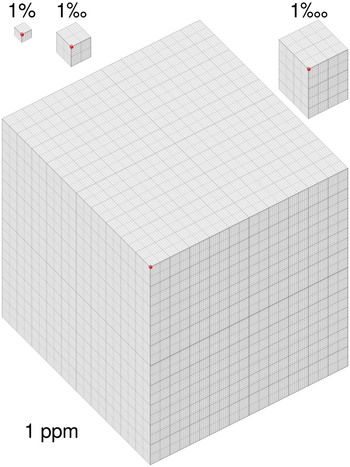# Concentration in parts per

(Redirected from Parts per million)Figure 1. Diagram showing percentage, parts per thousand, ten thousand and ppm. Click on image to see a larger version.

Pollutants can be problematic even in low concentrations, so it's necessary to be able to talk about very small amounts of a substance in a larger substance. This means it's useful to think of a total and how many parts of that total are a pollutant, or whatever scarce substance is being considered. If we divide a gas into a million parts, then the ppm is how many of those parts is the substance that we're interested in. In some situations it's important to distinguish between percent (or ppm, etc.) by mass, percent by volume, or percent by number. When not stated, amount by number is implied.

For example, the amount of carbon dioxide () in the atmosphere is now more than 400 parts per million (ppm), means that there are 400 molecules for every million molecules of air. Written another way, this is 0.4% a surprisingly small number, given that this is enough of a shift to cause climate change.

Amount Written as Number
percent % 0.01
part per thousand 0.001
part per ten thousand 0.000 1
part per million ppm 0.000 000 1
part per billion ppb 0.000 000 000 1

These are all dimensionless quantities used to describe the ratio of an amount of substance within another substance. For instance, 32 seconds would be roughly equivalent to one ppm of a year, and a millimeter is one ppm of a kilometer.

Ppm is commonly used to describe quantities of trace substances in order to communicate abundance; such as mercury in fish or the abundance of uranium within the Earth's crust.